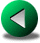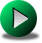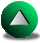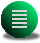Previous: Vorticity Budgets in a
Next: The Quasi-Geostrophic Model
Up: Finite Difference Methods in

# Vorticity Budgets in SW B-grid Models

This section stems from our interest in generalizing our experience from C-grid vorticity budgets to the B-grid (see Section 2.2.2). Under free-slip boundary conditions, the main difficulties arise from the fact that a prognostic equation for the tangential velocity along the wall has to be solved. This equation requires values of the pressure gradient along the wall, although the nearest elevation points are half a grid cell away in the interior. The zero-order solution is to use the same elevation value as at the nearest interior point, but this solution yields unreasonable shears of the tangential velocity close to the wall. In fact, the current along the wall tends to be zero or opposite to the overall gyre circulation. The problem stems from a poor representation of the geostrophic balance along the wall. For the current to be maximal at the wall, the pressure at the wall has to be larger than the pressure at the interior points. However, since the free-slip boundary condition yields a zero normal gradient for the tangential velocity, this means that the second derivative normal to the wall of the pressure should be approximately zero. Therefore, the pressure varies nearly linearly in the normal direction to the wall.

We therefore tested a simple linear extrapolation, using two interior pressure points. This approximation gives better results in the sense that there is no longer opposite currents along the wall. However the overall solution does not converge to the solution obtained with the C-grid model. After six years of simulation, the kinetic energy is three times as much as for the C-grid model (see Table 4.2). The circulation is too strong. From a vorticity budget perspective, the model cannot flux out the wind input of vorticity. The reason may come from the presence of a pressure term in the vorticity budget which acts as a torque4.1. This term should normally be zero. It arises from the non-cancellation of the pressure gradient terms close to each corner of the basin. This term is negative and therefore acts the same way as the wind input (see Table 4.2). It might be that the small discrepancy caused by the presence of this pressure term in the vorticity budget is enough that the model cannot converge to a reasonable solution. Nonetheless, we noted that the magnitude of this term decreases with resolution and might explain why the kinetic energy tends to decrease with increasing resolution. Due to the difficulty of tuning this free-slip B-grid model, we quickly gave up the idea of generalizing the experiments performed in the previous sections to the B-grid.

We noted however that some authors tackle the problem of the B-grid under free-slip boundary conditions. Hsieh et al. hsieh83, for instance, suggest the idea of shifting the whole grid, so that elevation points are on the boundary instead of velocity points. The trouble then is that we lose the main advantage of the traditional B-grid which is that the region for the vorticity budget domain is exactly the model domain. Using the traditional implementation of the B-grid, there is no advective flux of vorticity. More recently, Beckers beckers99 proposes to keep the traditional B-grid and to iterate at each time-step in order to get elevation values at land points close to the boundary that yield a zero normal velocity. This solution is of course more expensive. Unfortunately, we did not try to implement one of these solutions since our attention was already focused on more complex numerical methods. It is sufficient to note that implementation of free-slip boundary conditions are not trivial on B-grids. This adds to problems encountered with a similar implementation in a A-grid (see Section 3.4). Thus the free-slip boundary condition does not seem to be such an easy condition to implement in general in any model.

Table 4.2: Summary of the vorticity budget and kinetic energy diagnostics for the B-grid after a spin-up of 6 years. Instantaneous values after a 6 year spin-up. The model vorticity budget on a B-grid includes a pressure term due to the non-cancellation of the pressure gradient at the corners of the domain.
 Resolution K. Energy in 1010 m5s-2 FI in m2s-2 FO in m2s-2 Pressure term in m2s-2 20 km -- -- -- -- 10 km 2617 -0.2647 0.2639 -2.388 10-3 5 km 2473 -0.2606 0.2604 -1.238 10-3Previous: Vorticity Budgets in a
Next: The Quasi-Geostrophic Model
Up: Finite Difference Methods in

Frederic Dupont
2001-09-11